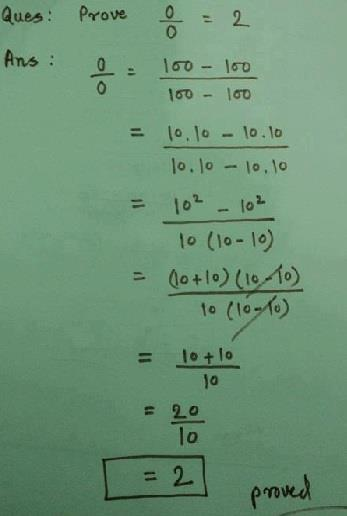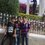# Fake ProvesAN ANYONE TELL ME THE MISTAKE IN IT....Note by Aßhĩмanyu Singh
6 years, 8 months ago

This discussion board is a place to discuss our Daily Challenges and the math and science related to those challenges. Explanations are more than just a solution — they should explain the steps and thinking strategies that you used to obtain the solution. Comments should further the discussion of math and science.

When posting on Brilliant:

• Use the emojis to react to an explanation, whether you're congratulating a job well done , or just really confused .
• Ask specific questions about the challenge or the steps in somebody's explanation. Well-posed questions can add a lot to the discussion, but posting "I don't understand!" doesn't help anyone.
• Try to contribute something new to the discussion, whether it is an extension, generalization or other idea related to the challenge.

MarkdownAppears as
*italics* or _italics_ italics
**bold** or __bold__ bold
- bulleted- list
• bulleted
• list
1. numbered2. list
1. numbered
2. list
Note: you must add a full line of space before and after lists for them to show up correctly
paragraph 1paragraph 2

paragraph 1

paragraph 2

[example link](https://brilliant.org)example link
> This is a quote
This is a quote
    # I indented these lines
# 4 spaces, and now they show
# up as a code block.

print "hello world"
# I indented these lines
# 4 spaces, and now they show
# up as a code block.

print "hello world"
MathAppears as
Remember to wrap math in $$ ... $$ or $ ... $ to ensure proper formatting.
2 \times 3 $2 \times 3$
2^{34} $2^{34}$
a_{i-1} $a_{i-1}$
\frac{2}{3} $\frac{2}{3}$
\sqrt{2} $\sqrt{2}$
\sum_{i=1}^3 $\sum_{i=1}^3$
\sin \theta $\sin \theta$
\boxed{123} $\boxed{123}$

Sort by:

You can't cancel $(10-10)$

- 6 years, 8 months ago

The problem is in the fourth step, where you have cancelled (10-10) with (10-10), because it would be the same as cancelling 0 with 0, so before performing the fourth step, try to prove 0/0 =1. Seems it is equal to both. But wait, try to do it with some variations equating it with 3,4,5 and it will satisfy all values. That why the solution is called indefinite because its solution set includes indefinitely many numbers. Can lead to a paradox!!!

- 6 years, 8 months ago

hey, if i say that prob. is in 1st step only like we know that 4/4=1 , 2/2 =1 , 3/3 =1, therefore 100-100/100-100 = 1 then

- 6 years, 8 months ago

Let me give you a reason for it, i have not seen it anywhere, just formulated it here only, so it can be wrong too.To start, I can write (100-100)/(100-100) as [100/(100-100)] - [100/(100-100)] which is equal to (100/0) - (100/0), it would be the same as infinity - infinity, and let me remind you that infinity is just a concept rather than a number so the solution set contains infinite numbers. You can ask if you have a doubt in this explanation.

- 6 years, 8 months ago

BUT Y IS 100-100/100-100 = 1 INCORRECT , IF WE DONT WRITE ANY FURTHER EXPLANATION

- 6 years, 8 months ago

100-100 can have infinite values, that is why mathematicians write it as not definite. If you know how to solve quadratic equations, u must know they have two roots, For example:- let us take a quadratic equation in which x=1 and x=2. but can i write 1=x=2? Obviously not! This is the case

- 6 years, 8 months ago

ohk

- 6 years, 8 months ago

thnxxx frnd

- 6 years, 8 months ago

ohk

- 6 years, 8 months ago

Aiya, the problem is the first step lah. You cannot divide anything by 0, otherwise you get a undefined result.

- 6 years, 8 months ago